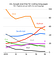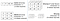# NumPy Basics: Machine Learning in PythonNumPy Logo Creator: Isabela Presedo-Floyd

Python is arguably the best programming language one can use to create Machine Learning and Artificial Intelligence Projects. Its simplicity, consistency, flexibility, platform independence and access to great libraries and frameworks makes it the first choice of an AI/ML Enthusiast to implement their vision.Image Source: Economist.com

To reduce the development time of a project, Python provides programmers almost 137,000 python libraries to work. A software library is pre-written code that developers use to solve common programming tasks. Few libraries that are commonly used in AI/ML projects are —

• Keras, TensorFlow, and Skikit-learn for Machine Learning
• NumPy for high-performance scientific computing and data analysis
• SciPy for advanced computing
• Pandas for general-purpose data analysis
• Seaborn and Matplotlib for data visualization

# NumPy: A tool for Arrays and Matrices in Python

NumPy short for Numerical Python, is one of the fundamental packages for Python providing support for large multidimensional arrays matrices along with a collection of high-level mathematical functions to execute these functions swiftly.
NumPy also forms the basis of other machine learning libraries like skikit-learn and SciPy. With its computational prowess directly comparable to other languages like C and Fortan, NumPy brings scientists even more advantage to use Python, a language much easier to learn and apply.

The simple answer is Vectorization of data.

Vectorization describes the absence of any explicit looping, indexing, etc., in the code. Rather than using these methods upfront, NumPy takes advantage of pre-compiled and highly optimized C code behind the scenes. This provides a much more concise and easier to read code.

Speed and Size are particularly important in scientific computing. Lets take a simple example to compare traditional python code to C code and NumPy code.
Lets take two simple 1-D array and write a code to multiply them in Python.

`Result = []for iter in range(len(array1)):    Result.append(array1[iter]*array2[iter])`

This produces the correct answer in the array Result, but if we have to use two arrays with millions of entries, we will have to face the inefficiency of Python. Python being an interpreted language, is slower than other major languages like C and Java. If we had to accomplish the same task in C, we would have a much faster result by writing:

`for(iter = 0; iter < rows; i++){    Result[iter] = array1[iter]*array2[iter];}`

This saves the overhead involved in interpreting Python code. Thus, to retain the benefits gained from coding in Python, we have to use NumPy. NumPy is able to reduce the lines of code while simultaneously using pre-compiled and optimized C code to execute element-by-element operation in a ndarray format.

`Result = array1*array2`

At its core, NumPy creates ndarray object. It encapsulates n-dimensional arrays of homogeneous data types and performs operation on compiled code for increased performance. Thus, operations like addition, multiplication, etc., that would require loops to operate on single numbers explicitly, can now be expressed in one single line of code.Image Source: pytolearn.csd.auth.gr

NumPy is majorly utilized for expressing images, sound waves, and other binary raw streams as an array of real numbers in n-dimension.

# Getting Started with NumPy

• You can install NumPy with:
Need Help? Readme.
`pip install numpy`
• Importing NumPy to your .py file:
`import numpyorimport numpy as nporimport numpy as <alias>`
• Creating Arrays:
`array1 = np.array([1,2,3]) #1-D Arrayarray2 = np.array([[1,2,3],[4,5,6]]) #2-D Arrayarray_dtype = np.array([1,2,3], dtype=<Data-Type>) #Data-Type is None by default.`
• Creating Arrays with Random elements:
`array_rand = np.random.randint(10,size=(3,4,5)) #Creates 3 arrays with 4 rows and 5 columns.`
• Selecting Elements:
`#Create a vector as a Rowvector_row = np.array([ 1,2,3,4,5,6 ])#Create a Matrixmatrix = np.array([[1,2,3],[4,5,6],[7,8,9]])print(matrix)#Select 3rd element of Vectorprint(vector_row)#Select 2nd row 2nd columnprint(matrix[1,1])#Select all elements of a vectorprint(vector_row[:])#Select everything up to and including the 3rd elementprint(vector_row[:3])#Select the everything after the 3rd elementprint(vector_row[3:])#Select the last elementprint(vector[-1])#Select the first 2 rows and all the columns of the matrixprint(matrix[:2,:])#Select all rows and the 2nd column of the matrixprint(matrix[:,1:2])`
• Describing a Matrix:
`#View the Number of Rows and Columnsprint(matrix.shape)#View the number of elements (rows*columns)print(matrix.size)#View the number of Dimensions(2 in this case)print(matrix.ndim)`
• Applying operations:
`#Create Matrix-1matrix_1 = np.array([[1,2,3],[4,5,6],[7,8,9]])#Create Matrix-2matrix_2 = np.array([[7,8,9],[4,5,6],[1,2,3]])#Add the 2 Matricesprint(np.add(matrix_1,matrix_2))#Subtractionprint(np.subtract(matrix_1,matrix_2))#Multiplication(Element wise, not Dot Product)print(matrix_1*matrix_2)`

Other functions of NumPy can be read here.

This blog provides a small overview of advantages and functionality of NumPy Library in Python. This documentation is by no means a complete guide to NumPy but a way to kickstart your journey of Machine Learning with NumPy.

Thanks for reading.
Don’t forget to click on 👏!

## Analytics Vidhya

Analytics Vidhya is a community of Analytics and Data…

## Analytics Vidhya

Analytics Vidhya is a community of Analytics and Data Science professionals. We are building the next-gen data science ecosystem https://www.analyticsvidhya.com

Written by

## Divyansh Chaudhary

Machine Learning and Python Student. Coding Enthusiast. Pursuing Bachelors in Computer Science.## Analytics Vidhya

Analytics Vidhya is a community of Analytics and Data Science professionals. We are building the next-gen data science ecosystem https://www.analyticsvidhya.com

Medium is an open platform where 170 million readers come to find insightful and dynamic thinking. Here, expert and undiscovered voices alike dive into the heart of any topic and bring new ideas to the surface. Learn more

Follow the writers, publications, and topics that matter to you, and you’ll see them on your homepage and in your inbox. Explore

If you have a story to tell, knowledge to share, or a perspective to offer — welcome home. It’s easy and free to post your thinking on any topic. Write on Medium